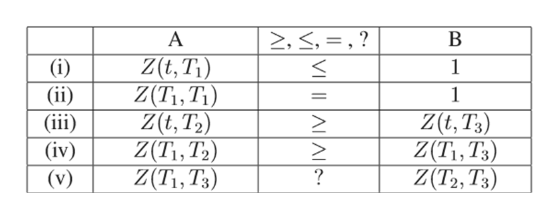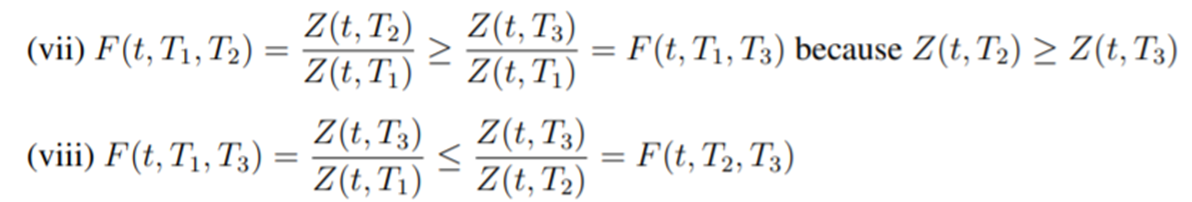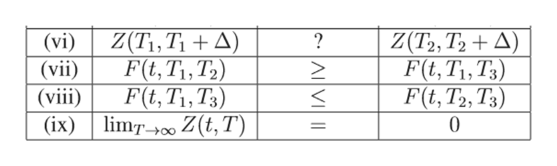Question 1. Suppose t ≤ T1 ≤ T2 ≤ T3, where t is the current time, and ∆ > 0. Recall that Z(T1, T2) is the price at time T1 of a ZCB with maturity T2 and F(T1, T2, T3) is the forward price at time T1 for a forward contract with maturity T2 on a ZCB with maturity T3. a) For each of the pairs of A and B in the table, choose the most appropriate relationship out of ≥, ≤, = , ?, where ? means the relationship is indeterminate. Give brief reasoning. A ≥, ≤, = , ? B (i) Z(t, T1) 1 (ii) Z(T1, T1) 1 (iii) Z(t, T2) Z(t, T3) (iv) Z(T1, T2) Z(T1, T3) (v) Z(T1, T3) Z(T2, T3) (vi) Z(T1, T1 + ∆) Z(T2, T2 + ∆) (vii) F(t, T1, T2) F(t, T1, T3) (viii) F(t, T1, T3) F(t, T2, T3) (ix) limT→∞ Z(t, T) 0 Hint: Remember that at current time t, F(t, ·, ·) is known but Z(T, ·) is a random variable. b) What can you say about interest rates between T1 and T2 if i) Z(t, T1) = Z(t, T2)? ii) Z(t, T1) > 0 and Z(t, T2) = 0?

Question

Question 1. Suppose t ≤ T1 ≤ T2 ≤ T3, where t is the current time, and ∆ > 0. Recall that Z(T1, T2) is the price at time T1 of a ZCB with maturity T2 and F(T1, T2, T3) is the forward price at time T1 for a forward contract with maturity T2 on a ZCB with maturity T3. a) For each of the pairs of A and B in the table, choose the most appropriate relationship out of ≥, ≤, = , ?, where ? means the relationship is indeterminate. Give brief reasoning. A ≥, ≤, = , ? B (i) Z(t, T1) 1 (ii) Z(T1, T1) 1 (iii) Z(t, T2) Z(t, T3) (iv) Z(T1, T2) Z(T1, T3) (v) Z(T1, T3) Z(T2, T3) (vi) Z(T1, T1 + ∆) Z(T2, T2 + ∆) (vii) F(t, T1, T2) F(t, T1, T3) (viii) F(t, T1, T3) F(t, T2, T3) (ix) limT→∞ Z(t, T) 0 Hint: Remember that at current time t, F(t, ·, ·) is known but Z(T, ·) is a random variable. b) What can you say about interest rates between T1 and T2 if i) Z(t, T1) = Z(t, T2)? ii) Z(t, T1) > 0 and Z(t, T2) = 0?

Step 1

Part (a)

Let's assume a continuous compoundde discount rate of r for the purpose of illustration.

Z (t, T1) = e-r(T1 – t) < 1

Please see the white board.

• Exponent of a negative number is always less than 1. This explains the answer to (i)
• If we put t = T1 in the equation above, we get Z (t = T1, T1) = e-r(T1 – T1) = 1. This explains answer to (ii)

• T2 ≤ T3; Hence, T2 – t ≤ T3 – t; Hence, - (T2 – t) ≥ - (T3 – t); Hence, Z (t, T2) = e-r(T2 – t) ≥ = e-r(T3 – t) = Z (t, T3). This explains the answer to (iii)

• Explnation to (iv) is same as that to (iii) above. Put t = T1 in the expression above and we end up getting what we are looking for in (iv)
• For (v) there will be no sure shot, certain relationship.help_outlineImage TranscriptioncloseА 2, <, = ,? В Z(t, T1) Z(T\,T1) Z(t,T2) Z(Ti, T2) Z(T1, T3) (i) (ii) (iii (iv) 1 1 Z(t, T3) Z(T\,T3) Z(T2, T3) ? (v) VI AA fullscreen
Step 2

(vi) the relationship is indeterminate. It can be either ways....help_outlineImage Transcriptionclose(vi) Z(T, T +A) (vii) Z(T, Т, + Д) F(t,T1, T3) F(t, T2, T3) ? F(t, T1, T2) F(t,Ti,T3) (viii) (ix) lim00 Z(t,T) 0 fullscreen

Want to see the full answer?

See Solution

Want to see this answer and more?

Our solutions are written by experts, many with advanced degrees, and available 24/7

See Solution
Tagged in